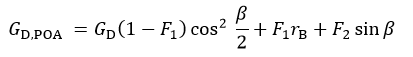# Perez Algorithm (Diffuse Sky)

1.) Find the factors that account for the respective angles of incidence of circumsolar radiation on the tilted and horizontal surfaces. The circumsolar radiation is considered to be from a point source.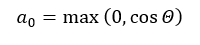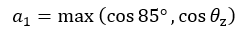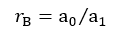2.) Compute the sky clearness as a function of the diffuse irradiance, direct normal irradiance, and zenith angle: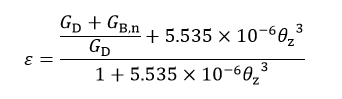3.) Find the brightness coefficients F1 and F2 from the lookup table in Figure 8 and the following equations: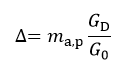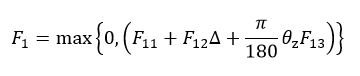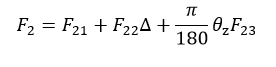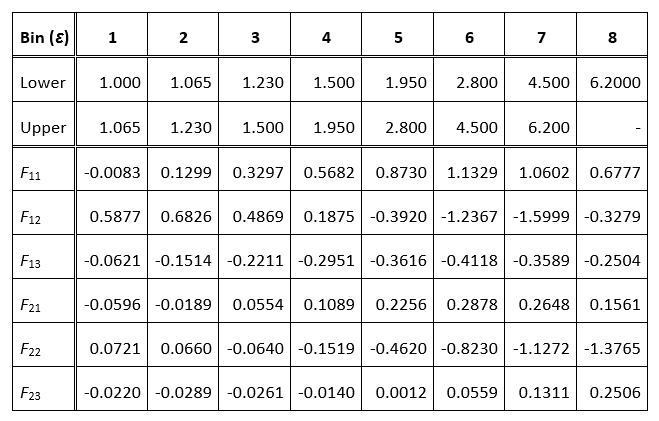### Figure 8. Coefficients of Slope Irradiance

4.) Compute the diffuse sky irradiance on the tilted plane: